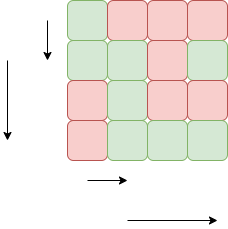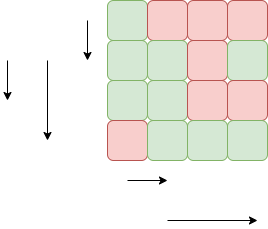# Rat in a Maze Problem when movement in all possible directions is allowed

Consider a rat placed at (0, 0) in a square matrix m[ ][ ] of order n and has to reach the destination at (n-1, n-1). The task is to find a sorted array of strings denoting all the possible directions which the rat can take to reach the destination at (n-1, n-1). The directions in which the rat can move are ‘U'(up), ‘D'(down), ‘L’ (left), ‘R’ (right).

Examples:

Input : N = 4
1 0 0 0
1 1 0 1
0 1 0 0
0 1 1 1
Output :
DRDDRRInput :N = 4
1 0 0 0
1 1 0 1
1 1 0 0
0 1 1 1
Output :
DDRDRR DRDDRR
Explanation:## Recommended: Please solve it on “PRACTICE” first, before moving on to the solution.

Solution:
Approach:

1. Start from the initial index (i.e. (0,0)) and look for the valid moves through the adjacent cells in the order Down->Left->Right->Up (so as to get the sorted paths) in the grid.
2. If the move is possible, then move to that cell while storing the character corresponding to the move(D,L,R,U) and again start looking for the valid move until the last index (i.e. (n-1,n-1)) is reached.
3. Also, keep on marking the cells as visited and when we traversed all the paths possible from that cell, then unmark that cell for other different paths and remove the character from the path formed.
4. As the last index of the grid(bottom right) is reached, then store the traversed path.

Below is the implementation of the above approach:

 `// C++ implemenattion of the above approach ` `#include ` `#define MAX 5 ` `using` `namespace` `std; ` ` `  `// Function returns true if the ` `// move taken is valid else  ` `// it will return false. ` `bool` `isSafe(``int` `row, ``int` `col, ``int` `m[][MAX],  ` `                 ``int` `n, ``bool` `visited[][MAX]) ` `{ ` `    ``if` `(row == -1 || row == n || col == -1 ||  ` `                  ``col == n || visited[row][col]  ` `                           ``|| m[row][col] == 0) ` `        ``return` `false``; ` ` `  `    ``return` `true``; ` `} ` ` `  `// Function to print all the possible ` `// paths from (0, 0) to (n-1, n-1). ` `void` `printPathUtil(``int` `row, ``int` `col, ``int` `m[][MAX],  ` `              ``int` `n, string& path, vector&  ` `               ``possiblePaths, ``bool` `visited[][MAX]) ` `{ ` `    ``// This will check the initial point ` `    ``// (i.e. (0, 0)) to start the paths. ` `    ``if` `(row == -1 || row == n || col == -1  ` `               ``|| col == n || visited[row][col]  ` `                           ``|| m[row][col] == 0) ` `        ``return``; ` ` `  `    ``// If reach the last cell (n-1, n-1) ` `    ``// then store the path and return ` `    ``if` `(row == n - 1 && col == n - 1) { ` `        ``possiblePaths.push_back(path); ` `        ``return``; ` `    ``} ` ` `  `    ``// Mark the cell as visited ` `    ``visited[row][col] = ``true``; ` ` `  `    ``// Try for all the 4 directions (down, left,  ` `    ``// right, up) in the given order to get the ` `    ``// paths in lexicographical order ` ` `  `    ``// Check if downward move is valid ` `    ``if` `(isSafe(row + 1, col, m, n, visited)) ` `    ``{ ` `        ``path.push_back(``'D'``); ` `        ``printPathUtil(row + 1, col, m, n, ` `                 ``path, possiblePaths, visited); ` `        ``path.pop_back(); ` `    ``} ` ` `  `    ``// Check if the left move is valid ` `    ``if` `(isSafe(row, col - 1, m, n, visited)) ` `    ``{ ` `        ``path.push_back(``'L'``); ` `        ``printPathUtil(row, col - 1, m, n, ` `                   ``path, possiblePaths, visited); ` `        ``path.pop_back(); ` `    ``} ` ` `  `    ``// Check if the right move is valid ` `    ``if` `(isSafe(row, col + 1, m, n, visited))  ` `    ``{ ` `        ``path.push_back(``'R'``); ` `        ``printPathUtil(row, col + 1, m, n, ` `                   ``path, possiblePaths, visited); ` `        ``path.pop_back(); ` `    ``} ` ` `  `     ``// Check if the upper move is valid ` `    ``if` `(isSafe(row - 1, col, m, n, visited)) ` `    ``{ ` `        ``path.push_back(``'U'``); ` `        ``printPathUtil(row - 1, col, m, n, ` `               ``path, possiblePaths, visited); ` `        ``path.pop_back(); ` `    ``} ` ` `  `    ``// Mark the cell as unvisited for  ` `    ``// other possible paths ` `    ``visited[row][col] = ``false``; ` `} ` ` `  `// Function to store and print ` `// all the valid paths  ` `void` `printPath(``int` `m[MAX][MAX], ``int` `n) ` `{ ` `    ``// vector to store all the possible paths ` `    ``vector possiblePaths; ` `    ``string path; ` `    ``bool` `visited[n][MAX]; ` `    ``memset``(visited, ``false``, ``sizeof``(visited)); ` `      `  `    ``// Call the utility function to ` `    ``// find the valid paths  ` `    ``printPathUtil(0, 0, m, n, path,  ` `                      ``possiblePaths, visited); ` ` `  `    ``// Print all possible paths ` `    ``for` `(``int` `i = 0; i < possiblePaths.size(); i++) ` `        ``cout << possiblePaths[i] << ``" "``; ` `} ` ` `  `// Driver code ` `int` `main() ` `{ ` `    ``int` `m[MAX][MAX] = { { 1, 0, 0, 0, 0 }, ` `                        ``{ 1, 1, 1, 1, 1 }, ` `                        ``{ 1, 1, 1, 0, 1 }, ` `                        ``{ 0, 0, 0, 0, 1 }, ` `                        ``{ 0, 0, 0, 0, 1 } }; ` `    ``int` `n = ``sizeof``(m) / ``sizeof``(m); ` `    ``printPath(m, n); ` ` `  `    ``return` `0; ` `} `

Output:

```DDRRURRDDD DDRURRRDDD DRDRURRDDD DRRRRDDD
```

Complexity Analysis:

• Time Complexity: O(3^(n^2)).
As there are N^2 cells from each cell there are 3 unvisited neighbouring cells. So the time complexity O(3^(N^2).
• Auxiliary Space: O(3^(n^2)).
As there can be atmost 3^(n^2) cells in the answer so the space complexity is O(3^(n^2)).

Attention reader! Don’t stop learning now. Get hold of all the important DSA concepts with the DSA Self Paced Course at a student-friendly price and become industry ready.

My Personal Notes arrow_drop_upCheck out this Author's contributed articles.

If you like GeeksforGeeks and would like to contribute, you can also write an article using contribute.geeksforgeeks.org or mail your article to contribute@geeksforgeeks.org. See your article appearing on the GeeksforGeeks main page and help other Geeks.

Please Improve this article if you find anything incorrect by clicking on the "Improve Article" button below.

Improved By : andrew1234

Article Tags :
Practice Tags :

9

Please write to us at contribute@geeksforgeeks.org to report any issue with the above content.